Courses

# Test: Deflection & Theories of Failure - 3

## 20 Questions MCQ Test Strength of Materials (SOM) | Test: Deflection & Theories of Failure - 3

Description
This mock test of Test: Deflection & Theories of Failure - 3 for Mechanical Engineering helps you for every Mechanical Engineering entrance exam. This contains 20 Multiple Choice Questions for Mechanical Engineering Test: Deflection & Theories of Failure - 3 (mcq) to study with solutions a complete question bank. The solved questions answers in this Test: Deflection & Theories of Failure - 3 quiz give you a good mix of easy questions and tough questions. Mechanical Engineering students definitely take this Test: Deflection & Theories of Failure - 3 exercise for a better result in the exam. You can find other Test: Deflection & Theories of Failure - 3 extra questions, long questions & short questions for Mechanical Engineering on EduRev as well by searching above.
QUESTION: 1

### What is the vertical displacement under the point of application of the force P?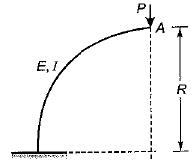Solution: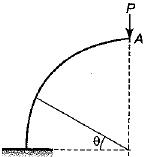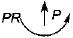M= PR - P(R - R cosθ)
M = PR cosθ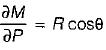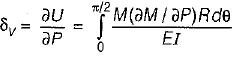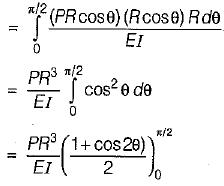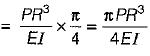QUESTION: 2

### A thin semicircular ring is hinged at each end and loaded by a central concentrated force, P as shown in figure. What is the horizontal reaction at each hinge?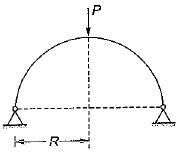Solution: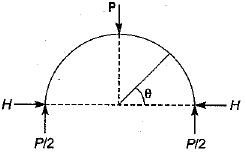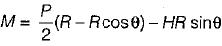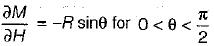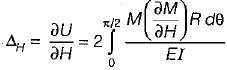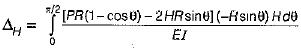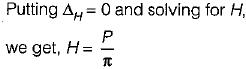QUESTION: 3

### Who enunciated the following theorem: Deflection of the point of application-of an external force acting on a beam is equal to the partial derivative of the work of deformation with respect to this force

Solution:
QUESTION: 4

Who enunciated the following theorem:
If unit loads rest upon a beam at the two points R and S, the deflection at R due to unit load at S equals the deflection at S due to the load at R

Solution:
QUESTION: 5

When a number of loads rest upon a beam the deflection at any point is equal to the sum of the deflections at this point due to each of the loads taken separately. This is according to

Solution:
QUESTION: 6

Total strain energy stored in a simply supported beam of span ‘L’ and flexural rigidity 'El' subjected to a concentrated load ‘W‘ at the center is equal to

Solution: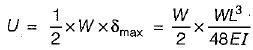∴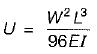QUESTION: 7

The strain energy stored in a shaft due to torsion is given by
Where V is the volume, G is modulus of rigidity and τs is the maximum shear stress on the surface of the shaft.

Solution:
QUESTION: 8

Which one of the following graphs represent Von Mises yield criterion?

Solution:

As per Von Mises yield criterion, the equation in the 2-D system is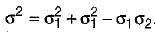.This equation represents an ellipse.

QUESTION: 9

Match the List-I with List-ll:
List-I
A. Maximum normal stress theory
B. Maximum principal strain theory
C. Maximum shear stress theory
List-ll
1. Mise's and Henkey’s theory
2. Rankine’s theory
3. St Venant’s theory
4. Guest’s Tresca’s theory
Codes:
A  B  C
(a) 4  1  2
(b) 2  3  4
(c) 3  2  4
(d) 2  3  1

Solution:
QUESTION: 10

Which of the following theories of failure is represented by square shape elastic boundary?

Solution:

Maximum principal stress theory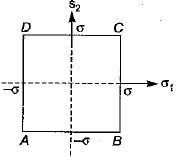QUESTION: 11

Principal stresses at a point in an elastic material are 100 MPa tensile 5 and 50 MPa tensile. What is the factor of safety against failure based on maximum shear strain energy theory? The elastic limit in simple tension is 173.2 MPa and Poisson’s ratio 0.3.

Solution:

1 - σ2)2 + (σ2 - σ3)2 + (σ3 - σ1)2 =  262
Here, σ1 = 100 MPa, σ2 = 50 MPa, σ3 = 0
∴ (100 - 50)2 + 502 + 1002 = 262
∴ σ2 = 7500 or σ = 86.6 MPa
Factor of safety,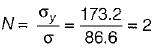QUESTION: 12

A uniform rod made of ductile material is subjected to tension. The value of octahedral shearing stress developed will be
where σy is the yield stress of ductile material.

Solution: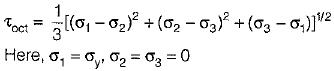∴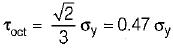QUESTION: 13

The value of octahedral shearing stress for a material subjected to hydrostatic pressure will be

Solution:

For hydrostatic pressure,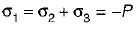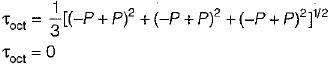QUESTION: 14

Which theory of failure is widely used in machine design dealing with ductile materials?

Solution:
QUESTION: 15

The ratio of the area under the bending moment diagram to the flexural rigidity between any two points along a beam gives the change in

Solution:
QUESTION: 16

A cantilever beam rectangular in cross-section is subjected to an isolated load at its free end. If the width of the beam is doubled, the deflection of the free end will be changed in the ratio of

Solution: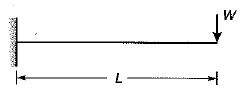initial width, b1 = b
final width, b2 = 2b
Deflection,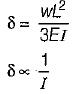But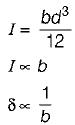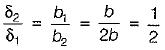QUESTION: 17

For a simply supported beam of length ‘L' subjected to downward load of uniform intensity w, Match List-I with List-II and select the correct answer using the codes given below the lists:
List-l
A. Slope of shear force diagram
B. Maximum shear force
C. Maximum deflection
D. Magnitude of maximum bending moment
List-II
1.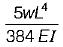2. w
3.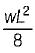4.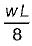Codes:
A  B  C  D
(a) 1  2  3   4
(b) 3  1  2   4
(c) 3  2  1   4
(d) 2  4  1   3

Solution:
QUESTION: 18

The Mohr’s circle of plane stress for a point in a body is shown. The design is to be done on the basis of the maximum shear stress theory for yielding. Then, yielding will just begin if the designer chooses a ductile material whose yield strength is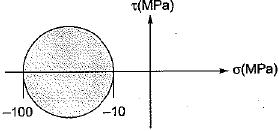Solution:

From maximum shear stress theory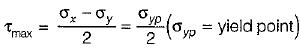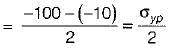σyp = -90 MPa

QUESTION: 19

As per the elastic theory of design, the factor of safety is the ratio of

Solution:

Usually ratio of ultimate stress to working stress is called factor of safety. In elastic theory of design the material factor of safety is only considered and the ratio of yield stress to working stress is called factor of safety.

QUESTION: 20

All the failure theories give nearly the same result

Solution:

When one of the principal stresses at a point is large in comparison to the other, the situation resembles uniaxial tension test. Therefore all theories give nearly same result.